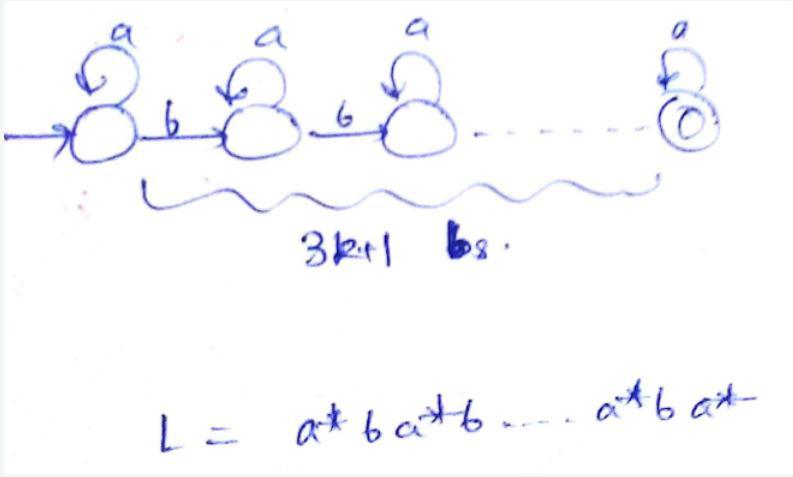GFG App
Open AppBrowser
Continue

# GATE | GATE-CS-2014-(Set-1) | Question 24

Which one of the following is TRUE?

(A)

The language L = {anbn | n ≥ 0} is regular.

(B)

The language L = {an| n is prime} is regular.

(C)

The language L = {w|w has 3k + 1 b’s for some k ∈N with Σ = {a, b}} is regular.

(D)

The language L = {ww | we Σ* with Σ = {0,1}} is regular.

Explanation:

(A) L = {a n b n |n >= 0} is not regular because there does not exists a finite automaton that can derive this grammar. Intuitively, finite automaton has finite memory, hence it can’t track number of as. It is a standard CFL though. (B) L = {a n b n |n is prime} is again not regular because there is no way to remember/check if current n is prime or not. Hence, no finite automaton exists to derive this grammar, thus it is not regular. (C) L = {w|w has 3k+1 bs} is a regular language because k is a fixed constant and we can easily emulate L as a ∗ ba ∗ …..ba ∗ such that there are exactly 3k + 1 bs and a ∗ s surrounding each b in the grammar.(D) L = {ww| w ∈ Σ ∗ } is again not a regular grammar, infact it is not even a CFG. There is no way to remember and derive double word using finite automaton. Hence, correct answer would be (C).

Quiz of this Question
Please comment below if you find anything wrong in the above post

My Personal Notes arrow_drop_up